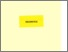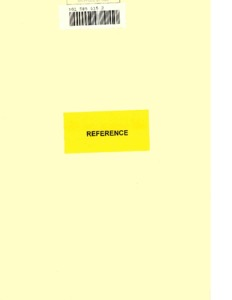# Spectral analysis of non-selfadjoint differential operators.

SABOORMALEKI, Mahmood. (1998). Spectral analysis of non-selfadjoint differential operators. Doctoral, Sheffield Hallam University (United Kingdom)..Preview
PDF (Version of Record)
10700952.pdf - Accepted Version

Related URLs:

## Abstract

This thesis is concerned with the extension of classical Titchmarsh-Weyl theory to non-selfadjoint Sturm-Liouville operators on the half-line. We introduce the thesis with some mathematical background which is needed for the development of the main results. This includes a brief summary of relevant aspects of Lebesgue measure and integration, analytic function theory, unbounded operator theory, and selfadjoint extensions of symmetric operators, and is given in the first two chapters. An introduction to Weyl theory and related topics for the self adjoint case can be found in Chapter 3. The main work on non-selfadjoint second order differential operators associated with the equation y" + qy = lambday begins in Chapter 4. We first describe Sims' extension of Weyl's limit point, limit circle theory to the non-selfadjoint case, and some later generalisations by McLeod. Some standard results of Titchmarsh are then extended from the selfadjoint to the non-selfadjoint case and an important result on the stability of the essential spectrum of a non-selfadjoint differential equation of form (py')'+qy =lambday is also obtained. In Chapter 5, we extend some results of Chaudhuri-Everitt to non-selfadjoint operators in which the potential satisfies the condition lim[x]infinityq(x) = L. Some worked examples at the end of Chapter 5 show that in certain cases where there is a complex boundary condition and real coefficient, or a complex coefficient with real boundary condition, some complex eigenvalues can be explicitly calculated. Finally in Chapter 6 we describe a physical problem which gives rise to a non-selfadjoint eigenvalue problem on the half-line. Keywords: Differential operator, Non-selfadjoint, Spectrum, Eigenvalue problem, Weyl m-function.

Item Type: Thesis (Doctoral) Thesis (Ph.D.)--Sheffield Hallam University (United Kingdom), 1998. Sheffield Hallam Doctoral Theses EPrints Services 10 Apr 2018 17:21 26 Apr 2021 12:21 https://shura.shu.ac.uk/id/eprint/20306

### Actions (login required)View Item